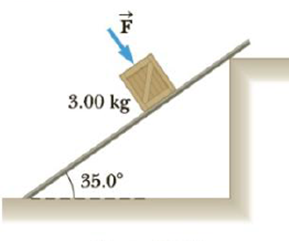Chapter 4, Problem 31P

Chapter
Section
Textbook Problem

The coefficient of static friction between the 3.00-kg crate and the 35.0° incline of Figure P4.31 is 0.300. What minimum force F → must be applied to the crate perpendicular to the incline to prevent the crate from sliding down the incline?Figure P4.31

To determine
The minimum force to be applied to the crate.

Explanation

Given info: The co-efficient of static friction ( μs ) is 0.300. The mass of the crate is 3.00 kg.

The free body diagram is given below.

From the above diagram,

N=F+mgcos35ο (I)

Fs=mgsin35ο (II)

• m is the mass of the car.
• g is the acceleration due to gravity.
• a is the acceleration.
• N is the normal force.
• F is the force applied.

The condition for the minimum force to be applied is,

FsμsN

On re-arranging,

FsNμs

Divide equation (II) by equation (I).

FsN=mgsin35οF+mgcos35ο (III)

For the applied force to be minimum,

FsN=μs (IV)

Equate equations (III) and (IV) to get minimum force ( Fmin )

Still sussing out bartleby?

Check out a sample textbook solution.

See a sample solution

The Solution to Your Study Problems

Bartleby provides explanations to thousands of textbook problems written by our experts, many with advanced degrees!

Get Started

Find more solutions based on key concepts